# Dinic算法（研究总结，网络流）

## 网络流最大流

### 网络流最大流的求解

1.找到一条从源点到汇点的路径，使得路径上任意一条边的残量>0（注意是小于而不是小于等于，这意味着这条边还可以分配流量），这条路径便称为增广路
2.找到这条路径上最小的F[u][v]（我们设F[u][v]表示u->v这条边上的残量即剩余流量），下面记为flow
3.将这条路径上的每一条有向边u->v的残量减去flow，同时对于起反向边v->u的残量加上flow（为什么呢？我们下面再讲）
4.重复上述过程，直到找不出增广路，此时我们就找到了最大流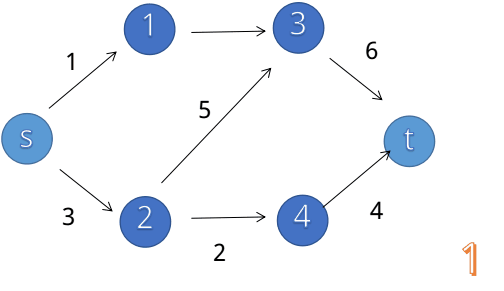### 为什么要连反向边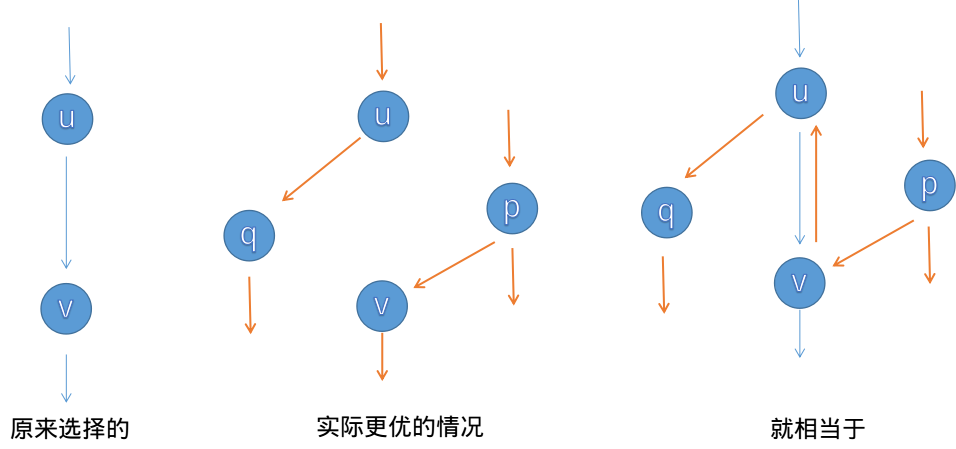### 朴素算法的低效之处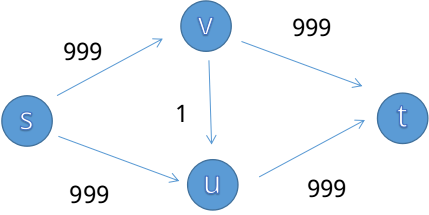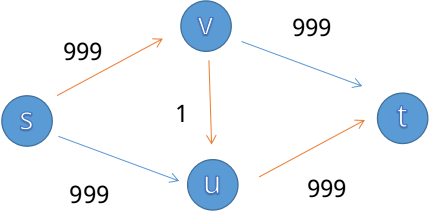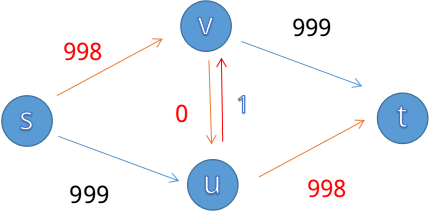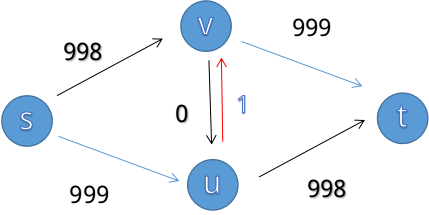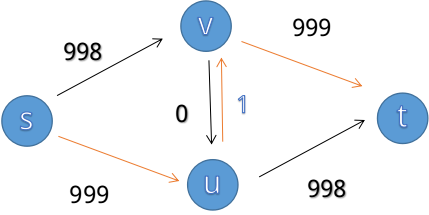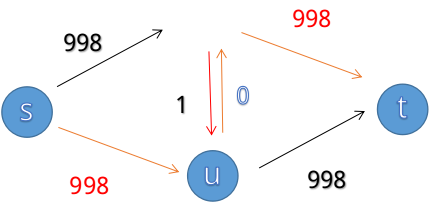## Dinic算法

int s,t;//源点和汇点
int cnt;//边的数量，从0开始编号。
int Next[maxM];//指向对应点的前一条边
int V[maxM];//每一条边指向的点
int W[maxM];//每一条边的残量
int Depth[maxN];//分层图中标记深度


Dinic主过程：

int Dinic()
{
int Ans=0;//记录最大流量
while (bfs())
{
while (int d=dfs(s,inf))
Ans+=d;
}
return Ans;
}


bfs分层图过程

bool bfs()
{
queue<int> Q;//定义一个bfs寻找分层图时的队列
while (!Q.empty())
Q.pop();
memset(Depth,0,sizeof(Depth));
Depth[s]=1;//源点深度为1
Q.push(s);
do
{
int u=Q.front();
Q.pop();
if ((W[i]>0)&&(Depth[V[i]]==0))//若该残量不为0，且V[i]还未分配深度，则给其分配深度并放入队列
{
Depth[V[i]]=Depth[u]+1;
Q.push(V[i]);
}
}
while (!Q.empty());
if (Depth[t]==0)//当汇点的深度不存在时，说明不存在分层图，同时也说明不存在增广路
return 0;
return 1;
}


dfs寻找增广路过程

int dfs(int u,int dist)//u是当前节点，dist是当前流量
{
if (u==t)//当已经到达汇点，直接返回
return dist;
{
if ((Depth[V[i]]==Depth[u]+1)&&(W[i]!=0))//注意这里要满足分层图和残量不为0两个条件
{
int di=dfs(V[i],min(dist,W[i]));//向下增广
if (di>0)//若增广成功
{
W[i]-=di;//正向边减
W[i^1]+=di;反向边加
return di;//向上传递
}
}
}
return 0;//否则说明没有增广路，返回0
}


class Graph
{
private:
int s,t;
int cnt;
int Next[maxM];
int V[maxM];
int W[maxM];
int Depth[maxN];
public:
int n;
void init(int nn,int ss,int tt)//初始化
{
n=nn;
s=ss;
t=tt;
cnt=-1;
memset(Next,-1,sizeof(Next));
return;
}
{
cnt++;
V[cnt]=v;
W[cnt]=w;
}
{
}
int dfs(int u,int dist)
{
//cout<<"Dfs:"<<u<<' '<<dist<<endl;
if (u==t)
return dist;
{
if ((Depth[V[i]]==Depth[u]+1)&&(W[i]!=0))
{
int di=dfs(V[i],min(dist,W[i]));
if (di>0)
{
W[i]-=di;
W[i^1]+=di;
return di;
}
}
}
return 0;
}
int bfs()
{
//cout<<"Bfs.begin:"<<endl;
queue<int> Q;
while (!Q.empty())
Q.pop();
memset(Depth,0,sizeof(Depth));
Depth[s]=1;
Q.push(s);
do
{
int u=Q.front();
//cout<<u<<endl;
Q.pop();
{
if ((W[i]>0)&&(Depth[V[i]]==0))
{
Depth[V[i]]=Depth[u]+1;
Q.push(V[i]);
}
}
}
while (!Q.empty());
//cout<<"Bfs.end"<<endl;
if (Depth[t]>0)
return 1;
return 0;
}
int Dinic()
{
int Ans=0;
while (bfs())
{
while (int d=dfs(s,inf))
Ans+=d;
}
return Ans;
}
};


## Dinic算法的优化

Dinic算法还有优化，这个优化被称为当前弧优化，即每一次dfs增广时不从第一条边开始，而是用一个数组cur记录点u之前循环到了哪一条边，以此来加速

class Graph
{
private:
int cnt;
int Next[maxM];
int W[maxM];
int V[maxM];
int Depth[maxN];
int cur[maxN];//cur就是记录当前点u循环到了哪一条边
public:
int s,t;
void init()
{
cnt=-1;
memset(Next,-1,sizeof(Next));
}
{
cnt++;
V[cnt]=v;
W[cnt]=w;
}
{
}
int dfs(int u,int flow)
{
if (u==t)
return flow;
for (int& i=cur[u];i!=-1;i=Next[i])//注意这里的&符号，这样i增加的同时也能改变cur[u]的值，达到记录当前弧的目的
{
if ((Depth[V[i]]==Depth[u]+1)&&(W[i]!=0))
{
int di=dfs(V[i],min(flow,W[i]));
if (di>0)
{
W[i]-=di;
W[i^1]+=di;
return di;
}
}
}
return 0;
}
int bfs()
{
queue<int> Q;
while (!Q.empty())
Q.pop();
memset(Depth,0,sizeof(Depth));
Depth[s]=1;
Q.push(s);
do
{
int u=Q.front();
Q.pop();
if ((Depth[V[i]]==0)&&(W[i]>0))
{
Depth[V[i]]=Depth[u]+1;
Q.push(V[i]);
}
}
while (!Q.empty());
if (Depth[t]>0)
return 1;
return 0;
}
int Dinic()
{
int Ans=0;
while (bfs())
{
for (int i=1;i<=n;i++)//每一次建立完分层图后都要把cur置为每一个点的第一条边 感谢@青衫白叙指出这里之前的一个疏漏